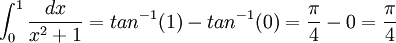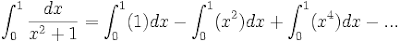## Tuesday, November 27, 2007

### π/4 = 1 - 1/3 + 1/5 - 1/7 + ...

In 1675, Gottfried Leibniz was able to show the following Theorem:

Theorem: π/4 = 1 - 1/3 + 1/5 - 1/7 + ...

Proof:

(1) D(tan-1x)= 1/(x2 + 1) [See Theorem, here]

(2) So:

∫ dx/(x2 + 1) = tan-1 x [See Theorem 2, here]

(3) Since tan(0) = 0 and tan(π/4) = 1, we have:(4) If we assume that (x2 + 1) ≠ 0, then we have:

1/(x2 + 1) = 1 - x2 + x4 - x6 + x8 - ...

since:

(a) Let a = 1 - x2 + x4 - x6 + x8 - ...

(b) Then:

x2*a = x2 - x4 + x6 - x8 + ...

(c) Then:

a + x2*a = 1

(d) a + x2*a = a(1 + x2) = 1

(e) So:

a = 1/(1 + x2)

which means that:

1/(1 + x2) = 1 - x2 + x4 - x6 + x8 - ...

(5) But this means that:(6) Further, we know that:1 - 1/3 + 1/5 - 1/7 + ...

(7) Putting step #3 together with step #5 and step #6 gives us:

π/4 = 1 - 1/3 + 1/5 - 1/7 + ...

QED

References
• Jean-Pierre Tignol, Galois' Theory of Algebraic Equations, World Scientific, 2001